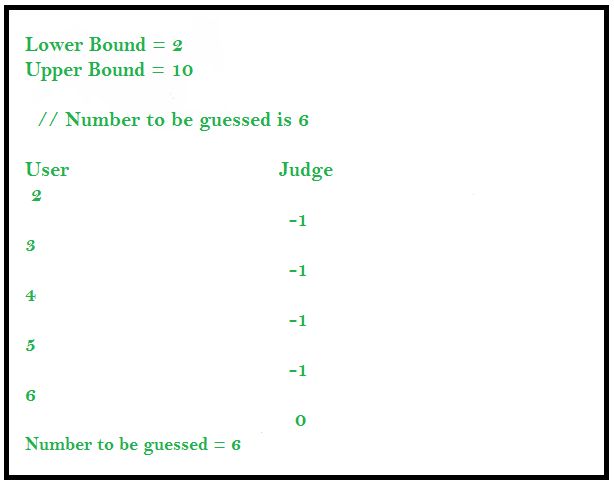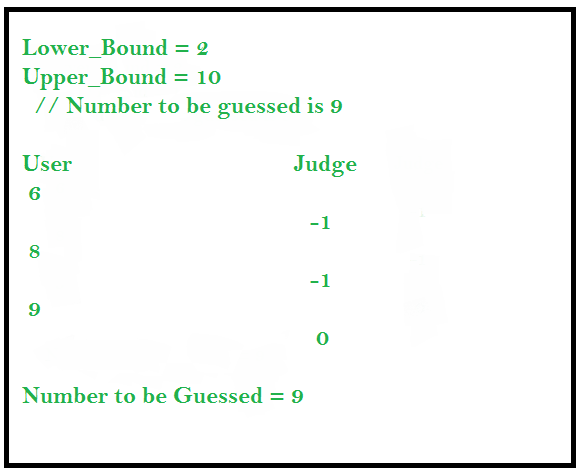# Interactive Problems in Competitive Programming

Interactive Problems are those problems in which our solution or code interacts with the judge in real time. When we develop a solution for an Interactive Problem then the input data given to our solution may not be predetermined but is built for that problem specifically. The solution performs a series of exchange of data with the judge and at the end of the conversation the judge decides whether our solution was correct or not.

## Guessing the Number (An Interactive Problem)

In this problem the user has to guess the number during a communication with the judge. The user is provided with the upper and lower bound and he/she can ask the judge whether a number is the number to be guessed. The judge replies with -1 if the number is smaller than the number to be guessed or 1 if number is greater than the number to be guessed or 0 if it is equal to the number to be guessed.

### Approach 1 : Linear Guessing

The user can query the judge for all the numbers between lower limit and upper limit to find the solution.`import` `java.util.*; ` `class` `GFG { ` `    ``public` `static` `void` `main(String[] args) ` `    ``{ ` `        ``Scanner sc1 = ``new` `Scanner(System.in); ` `        ``int` `lower_bound = ``2``; ` `        ``int` `upper_bound = ``10``; ` ` `  `        ``// Number to be guessed is 6 ` ` `  `        ``// Iterating from lower_bound to upper_bound ` `        ``for` `(``int` `i = lower_bound; i <= upper_bound; i++) { ` `            ``System.out.println(i); ` ` `  `            ``// Input the response from the judge ` `            ``int` `response = sc1.nextInt(); ` ` `  `            ``if` `(response == ``0``) { ` `                ``System.out.println(``"Number guessed is :"` `+ i); ` `                ``break``; ` `            ``} ` `        ``} ` `    ``} ` `} `

Time Complexity: O(n)

### Approach 2 : Applying Binary Search

We can also apply binary search interactively to find the solution. This solution is efficient as compared to the previous approach.`import` `java.util.*; ` `class` `GFG { ` `    ``public` `static` `void` `main(String[] args) ` `    ``{ ` `        ``Scanner sc1 = ``new` `Scanner(System.in); ` `        ``int` `lower_bound = ``2``; ` `        ``int` `upper_bound = ``10``; ` ` `  `        ``// Number to be guessed is 9 ` ` `  `        ``// Applying Binary Search interactively ` `        ``while` `(lower_bound <= upper_bound) { ` `            ``int` `mid = (lower_bound + upper_bound) / ``2``; ` ` `  `            ``// Print the guessed number ` `            ``System.out.println(mid); ` ` `  `            ``// Input the response from the judge ` `            ``int` `response = sc1.nextInt(); ` ` `  `            ``if` `(response == -``1``) { ` `                ``lower_bound = mid + ``1``; ` `            ``} ` `            ``else` `if` `(response == ``1``) { ` `                ``upper_bound = mid - ``1``; ` `            ``} ` `            ``else` `if` `(response == ``0``) { ` `                ``System.out.println(``"Number guessed is :"` `+ mid); ` `                ``break``; ` `            ``} ` `        ``} ` `    ``} ` `} `

Time Complexity: O(logn)My Personal Notes arrow_drop_upCheck out this Author's contributed articles.

If you like GeeksforGeeks and would like to contribute, you can also write an article using contribute.geeksforgeeks.org or mail your article to contribute@geeksforgeeks.org. See your article appearing on the GeeksforGeeks main page and help other Geeks.

Please Improve this article if you find anything incorrect by clicking on the "Improve Article" button below.